# Application of ordinary light-emitting diode in power indicator

There are many kinds of diodes, and there are many kinds of light-emitting diodes. There are plug-ins and patches. Light-emitting diodes are often used as display indicators. Therefore, many power indicator lights use light-emitting diode display methods, such as our product indicator lights, Switch socket power indication, etc.

The following uses a common light-emitting diode 333-2SURD/S530-A3 as an example to illustrate the role of this LED in the power indicator light. The size of this LED is Ф5MM, standard LED through hole.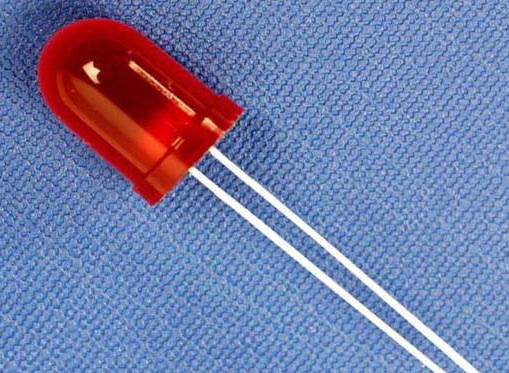Maximum forward current IF=25mA, maximum reverse voltage Vr=5V, forward working voltage Vf=1.7V, as shown in the following table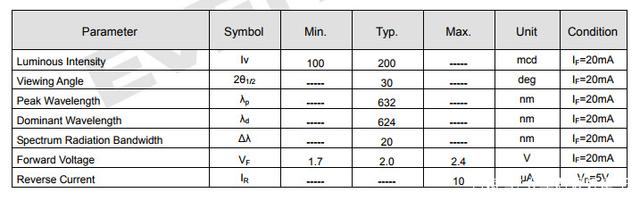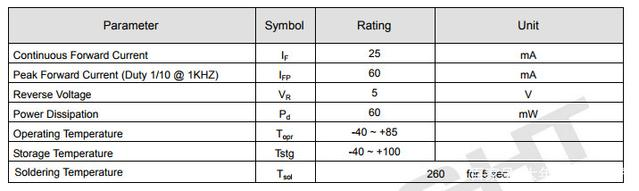# 1. In the DC power indicator

This kind of DC power supply circuit is relatively simple and can be driven directly. It only needs to add a current limiting resistor, and it can also be scanned. It should be noted that the current-limiting resistance R≥(5V-Vf)/IF, Vf is the forward voltage drop, IF is the operating current, where R should be at least 132Ω, here 1K ohm, the current is 5mA, the limit The current resistance cannot be too large, otherwise it will affect the brightness.# 2. AC power indicator

In the case of AC input, the simpler circuit is to directly connect a current limiting resistor in series. Although this circuit is simple and the cost is very low, the power consumption of the resistor is very large, so the selection of resistor power is a problem. At this time, the current limiting resistor R ≥220V/IF, because it is only used as an indicator, the current will not be set very large, here R1 selects 51K, then the current is about 4.3mA, the power consumed on R reaches nearly 1W, you can choose a 2W resistor, D1 role is Reverse protection LED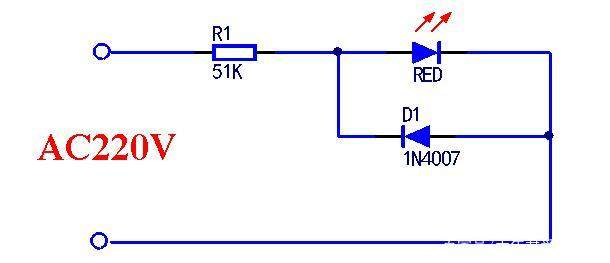It can also be connected in this way, adopting the half-wave working mode, the LED will be lit in half a week, and D2 reverse protectionIf conditions permit, you can use a RC step-down circuit, so that the power consumption of the resistor is not so large, the output current I=0.5|I|=34500C, where C is the capacity of the capacitor C1, calculated at 5mA, then choose the capacitor size is 0.15uF Just left and right, R1 is the discharge resistance.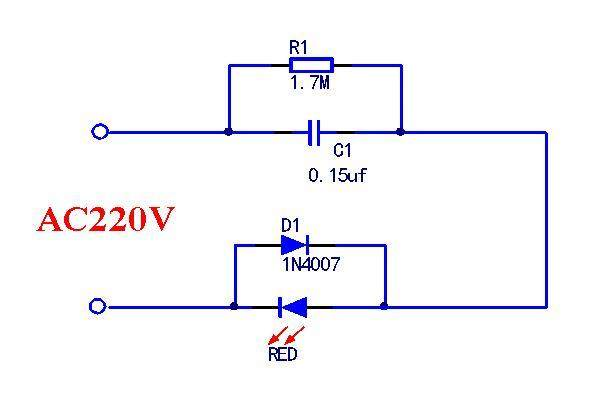PREVIOUS:None NEXT:None